Greatest Common Factor (GCF) MathBitsNotebook.com Terms of Use   Contact Person: Donna Roberts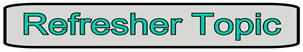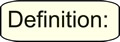When two numbers (expressions) are multiplied, the answer is called a product. The numbers (expressions) that were multiplied are called the factors. Numeric Example: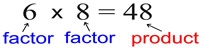Algebraic Example: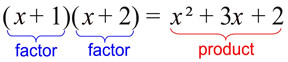The greatest common factor, GCF, is the largest number (expression) that divides exactly into two or more numbers (expressions). The greatest common factor will prove to be an important value. The first step in every factoring problem is to determine and factor out the greatest common factor.
Let's look at some examples of finding the greatest common factor, GCF.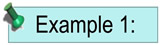Find the greatest common factor of 12, 24, and 42.For help with GCF on your calculator, Click Here!
Solution:
Factors of 12: 2,3,4,6,12
Factors of 24: 2,3,4,
6,8,12,24
Factors of 42: 2,3,6,7,21,42
Greatest Common Factor = 6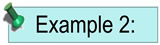Find the GCF of x3, x2 and x5.
Solution:
The highest power of x that is common to all three of the terms is x2.
Greatest Common Factor = x2.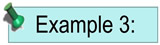Find the GCF of 3a3b4, 6a4b2, and 12a6b.
Solution:
The highest power of a that is common to all three of the terms is a3.
The highest power of b that is common to all three of the terms is b.
The GCF of the coefficients 3, 6 and 12, is 3.
Greatest Common Factor = 3a3b.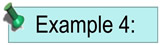Find the GCF of the following terms:
3(x + 1)3, 9(x + 1)2, and 12(x + 1).
Solution:
The GCF of 3, 9 and 12 is 3.
The highest power of (x + 1) is (x + 1)1.
Greatest Common Factor = 3(x + 1).